by Tom Xu
I'm an expert in Search Engine Optimization (SEO) and digital marketing. I write about different blog topics with an fo...

# How to Do Matrix MultiplicationImage by ARENA Creative on Shutterstock

Linear algebra can be tough to learn. However, it’s critical that you understand it, instead of simply memorizing how each operation in linear algebra is performed.

Understanding why and how the operations are performed in linear algebra requires logic and practice. Matrix multiplication is just one of the operations covered when studying linear algebra in college, and understanding how to perform this operation by hand is required for exams.

Let’s look at how to perform matrix multiplication between a matrix and a scalar number, vector, or matrix.

## What is matrix multiplication?

Linear algebra is a relatively new field of study in mathematics. In 1812, French mathematician Jacques Philippe Marie Binet was the first to describe matrix multiplication. However, some scholars question this date since the first matrix wasn’t fully described until 1857 by Arthur Cayley in Britain.

For perspective on how “young” linear algebra is, the Pythagorean theorem in geometry was described between 400 and 800 BCE.

A matrix is a rectangular array of numbers or expressions. It is arranged in rows and columns. The number of rows is represented by “m” and the number of columns is represented by “n.”

Each element in the matrix can be represented by “ai,j” where “j” increases with respect to the column and “i” increases with respect to the number of rows, as you can see in the figure below.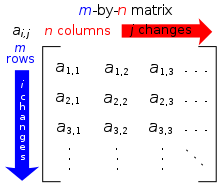Photo Credit

## How to multiply a matrix by a number

Multiplying a matrix by a number is straightforward. Simply, each element in the matrix is multiplied by the number. The strategy doesn’t change based on the number of rows and columns.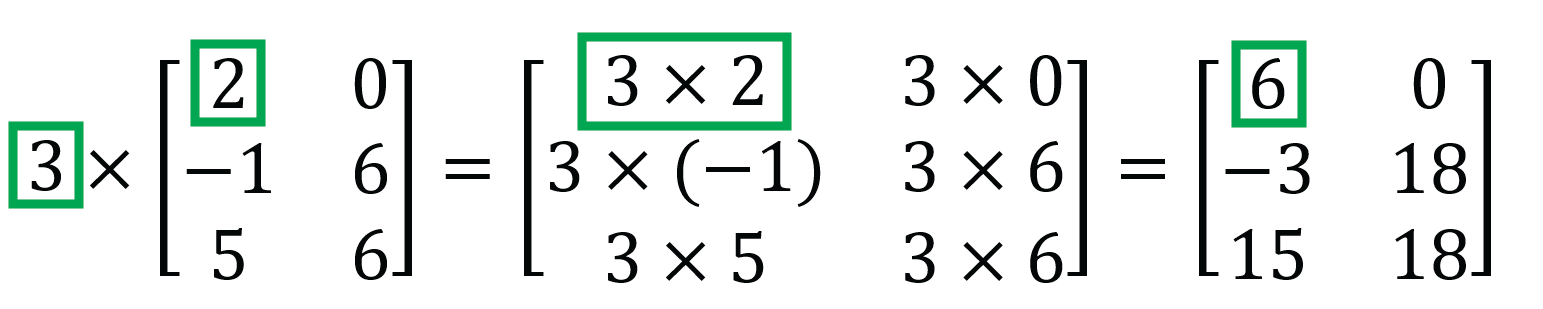When a number is multiplied by a matrix, this is called scalar multiplication. A scalar is a quantity that is fully described by a single magnitude or numerical value. In the example above, 3 is a scalar.

## How to multiply a matrix by a matrix

Multiplication between matrices is more complex. While calculating the result of matrix multiplication by hand is likely impractical in the real world, understanding the process will deepen your understanding of linear algebra.

When multiplying a matrix by a matrix, the number of columns in the first matrix must equal the number of rows in the second matrix, otherwise the operation cannot be defined.

The result will be a matrix with m columns and p rows. This is represented by the following equation:

[mn ][ np] [mp]

To calculate the result of a matrix multiplied by a matrix, calculate the “dot product” of each row and column.

A dot product is an algebraic operation where two lists of numbers (vectors) that are equal in length return a single number. A dot product is also called a scalar product as the calculation will result in a single number.

Matrices of different sizes can be multiplied together. Here, we have matrix A with “m” rows and “n” columns, and matrix B with “n” rows and “p” columns.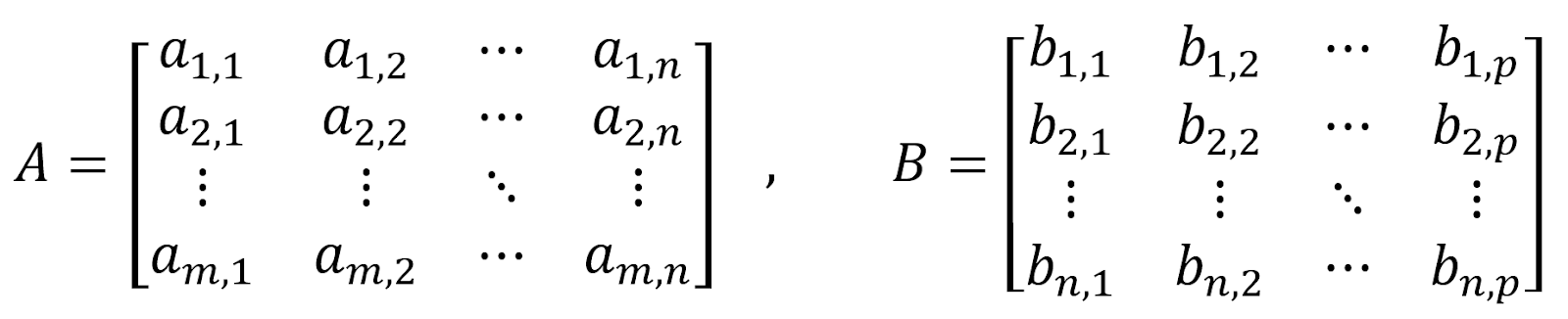The resulting matrix product is the matrix C with “m” rows and “p” columns.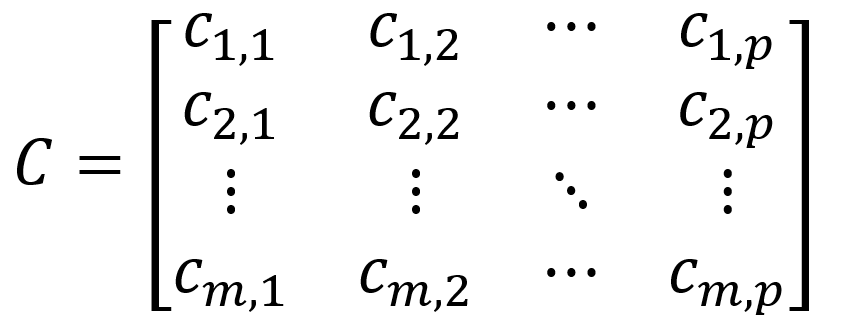To calculate each element in matrix C, the below formulas are used.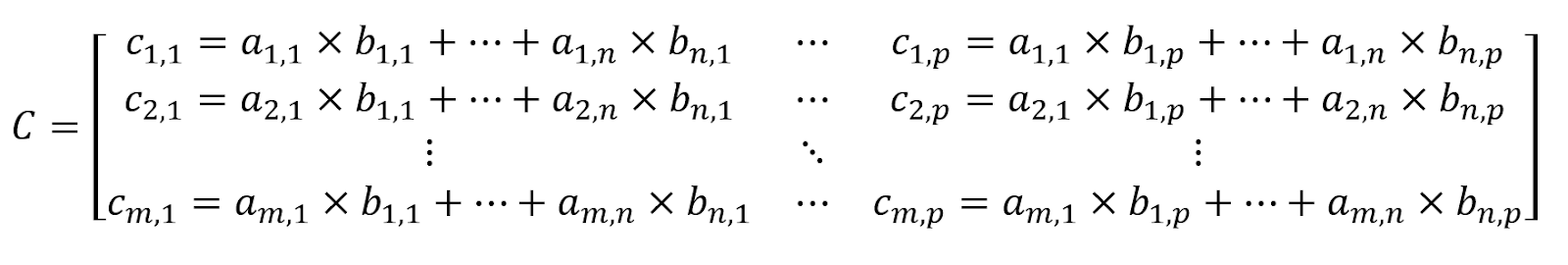Let’s do an example. Here, we have a matrix with 2 rows and 3 columns multiplied by a matrix with 3 columns and 2 rows. The result will be a matrix with 2 rows and 2 columns.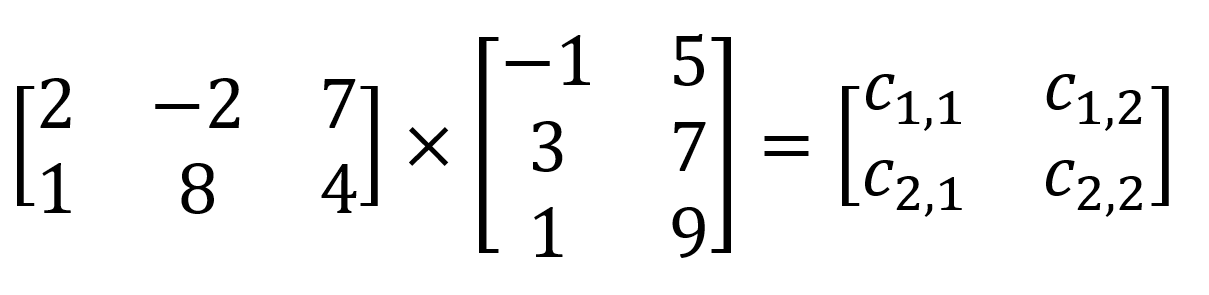To calculate c1,1, we must determine the dot product of the 1st row in the 1st matrix and the 1st column of the 2nd matrix.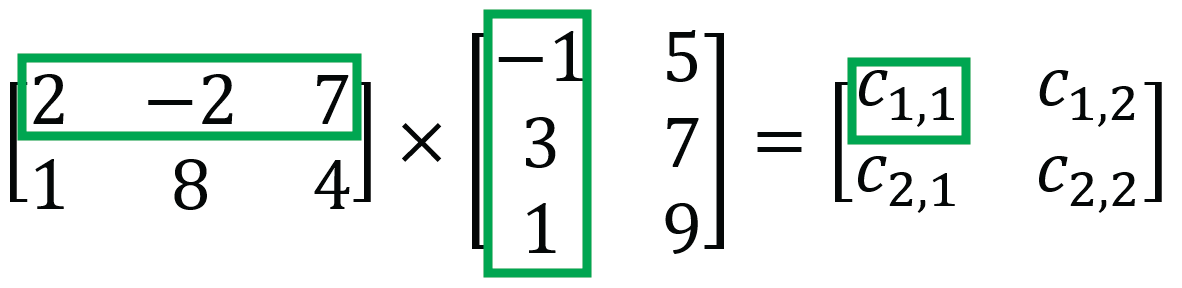The formula is as follows:

c1,1=2 (-1) +(-2) 3 +7 1 =-1

Likewise, c1,2 is calculated by determining the dot product of the 1st row and the 2nd column of the 2nd matrix.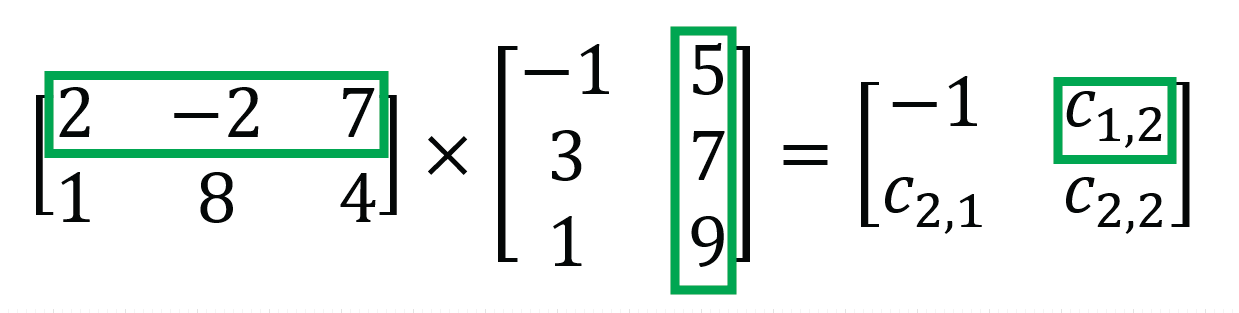The formula is as follows:

c1,2=2 5 +(-2)7 +7 9 =59

The following equations are used to calculate c2,1 and c2,2:

c2,1=1 (-1) +8 3 +4 1 =27

c2,2=1 5 +8 7 +4 9 =97

The matrix-matrix product is: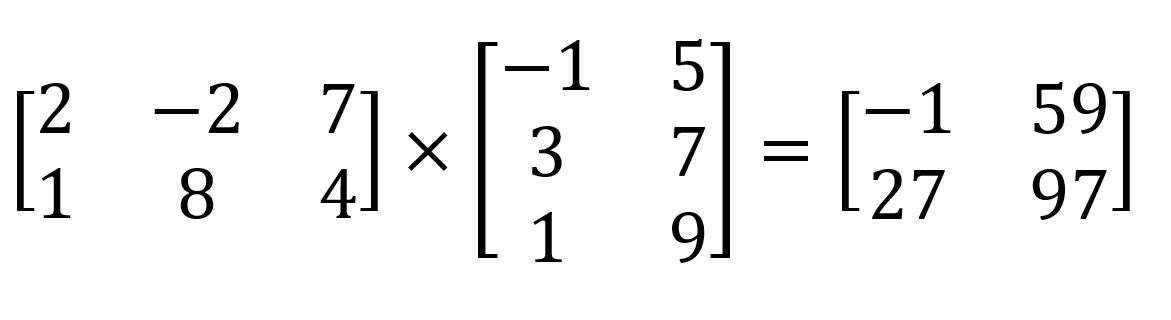## How to multiply a matrix by a vector

A vector is a matrix with only one row or only one column. Multiplying a matrix by a vector follows a similar procedure when multiplying a matrix by a matrix.

Here we have a vector, x=(4,-2,1), and are multiplying it by the matrix A.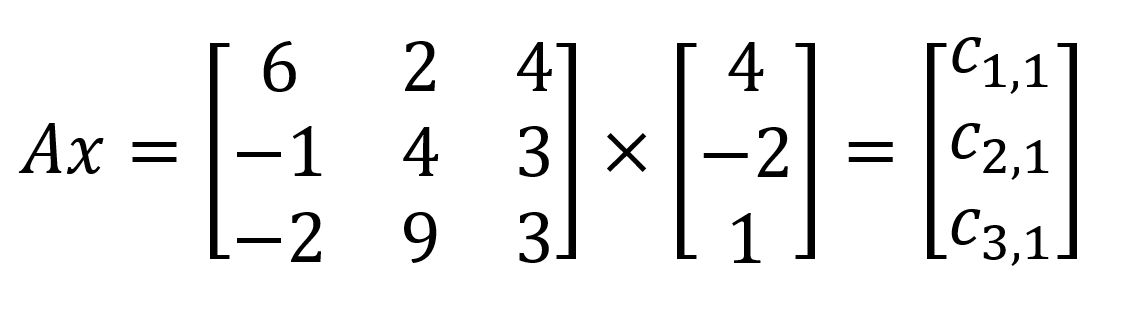Calculate c1,1 by determining the dot product of the 1st row multiplied by the vector.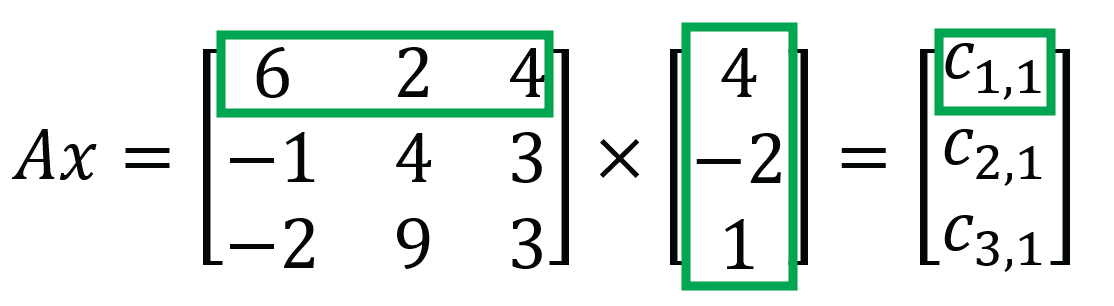See the equation below to calculate c1,1.

c1,1=64 +2(-2) +41=24

Likewise, here are the equations to calculate c2,1 and c3,1.

c2,1 =-14 +4(-2) +31 =-9

c3,1 =-24 +9(-2) +31 =-23

The solution to the matrix-vector multiplication is as follows: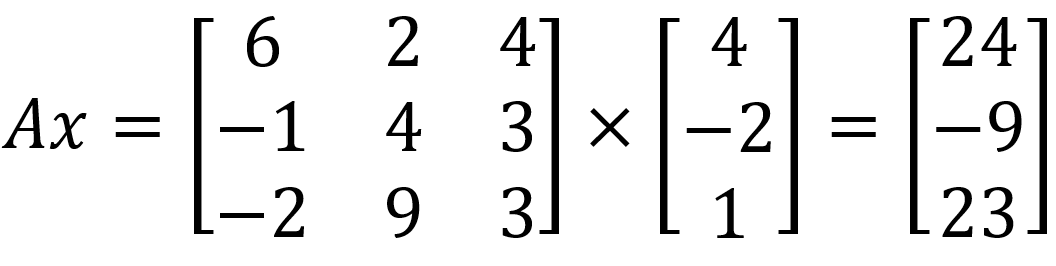Overall, matrix-vector multiplication follows the same procedure as matrix-matrix multiplication. However, it’s important to note the naming conventions.

## What is the order of operations?

Unlike multiplication between real numbers, matrix multiplication is not commutative. As in, the result does not depend on the order of the elements.

For example, in the below calculation:

123=312=36

Both sides of the equation equal 36 no matter the order of the numbers. This is not true of all matrices. Commutative matrices occur when a matrix is multiplied by itself, or when it is multiplied by the identity matrix.

For example, we have matrix A multiplied by matrix B with matrix C as a result.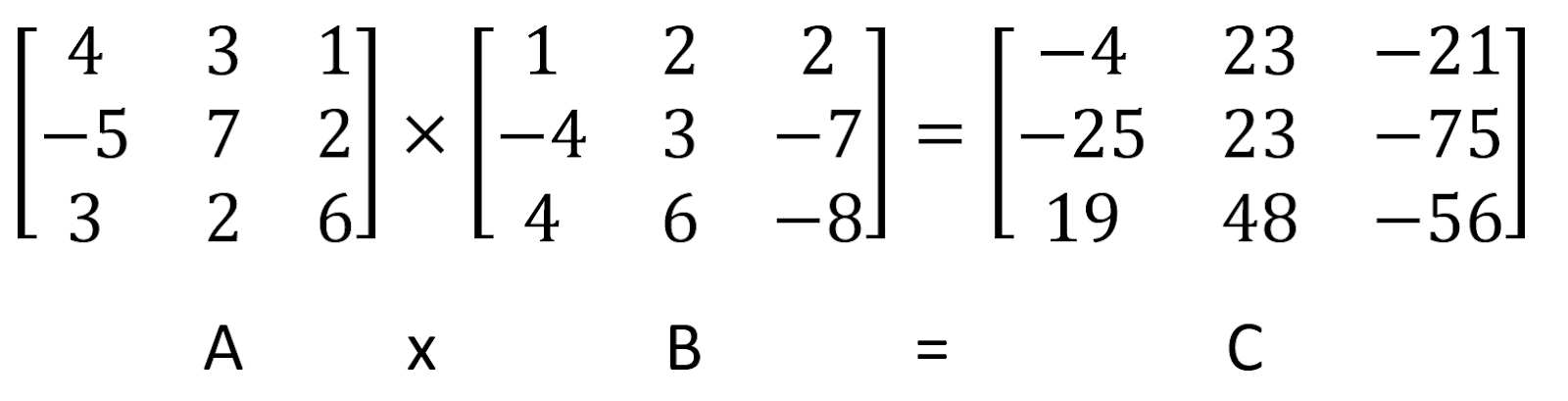But if we have matrix B multiplied by matrix A, the end result is a different matrix D.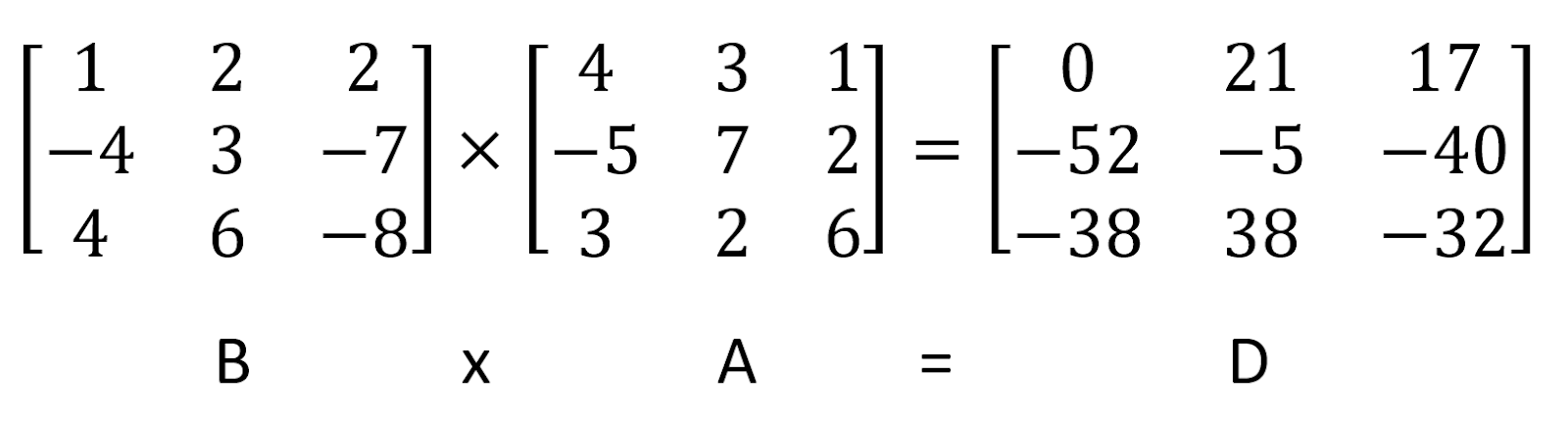Let’s compare the calculation for the first dot product to understand why there is a difference.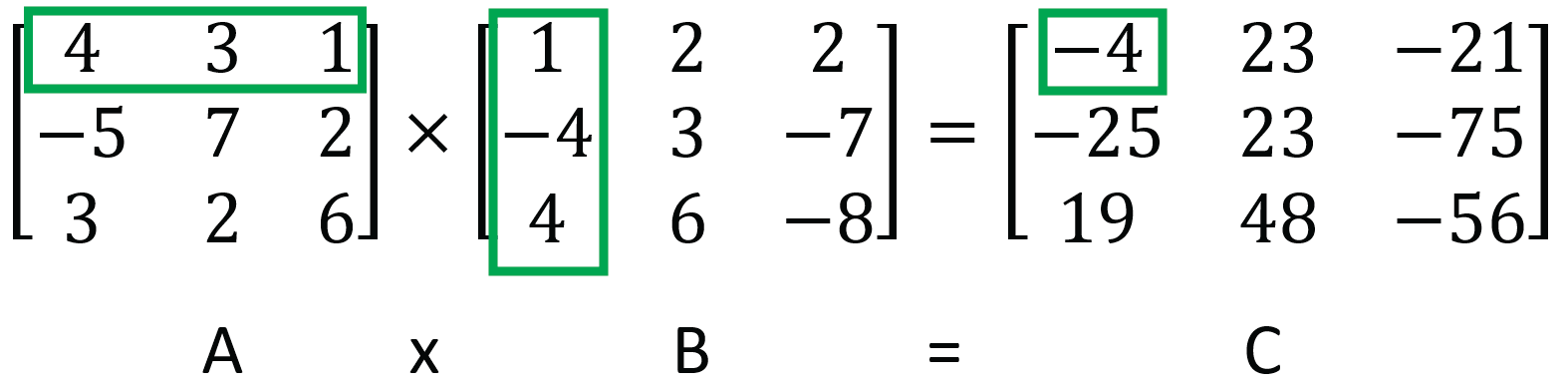Calculating the first value for c1,1 uses the formula:

c1,1=41 +3(-4) +14=-4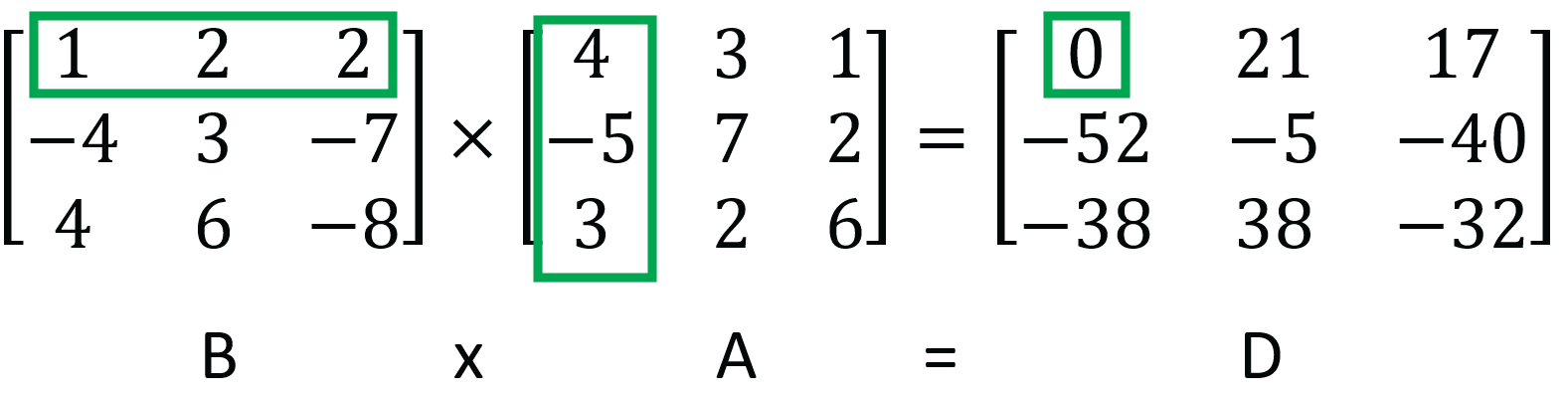d1,1= 14 +2(-5) +23 =0

It’s clear from the way the dot product is calculated that order matters when multiplying matrices. Notice how different numbers are highlighted in the matrices when the order of the matrix multiplication is switched.

## How to apply matrix multiplication to real problems

Matrix multiplication has applications in the real world, even if we might not think of these situations as matrix multiplication.

For example, matrix multiplication can be used to calculate the profit of a store.

The below table corresponds to the cost of each item for sale in a clothing store.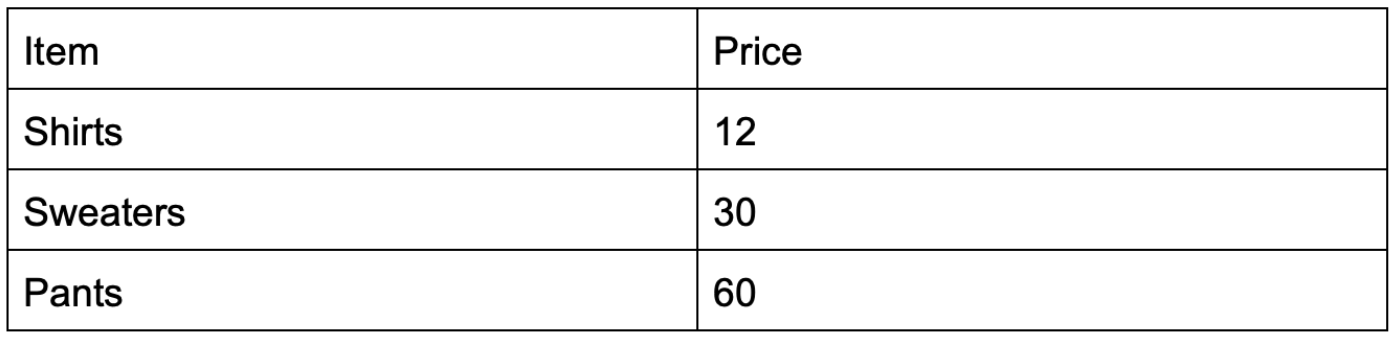The table below represents how many shirts, sweaters, and pants were sold on Monday, Tuesday, and Wednesday.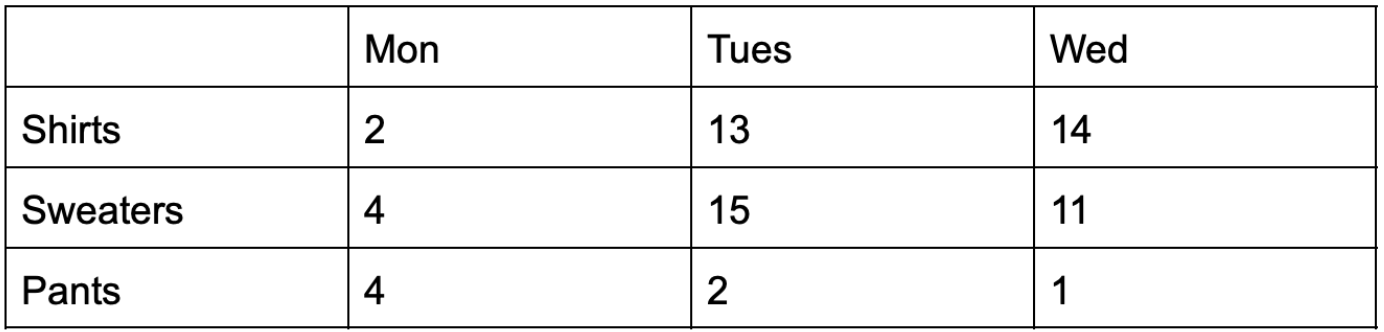The profit on Monday can be calculated with the below equation.

Monday Profit=Price of ShirtShirts Sold+Price of SweaterSweaters Sold

+Price of PantsPants Sold

=122 + 304 + 604 =384

Look familiar? We’ve just calculated the dot product for Monday sales.

Let’s represent this data with a matrix and a vector.After matrix multiplication, we can calculate the sales on Monday, Tuesday, and Wednesday below.

The below equation can be used to calculate the profit on Tuesday.

Tuesday profit = c2,1=412 +1530 +1160 =1158

Likewise, the profit on Wednesday can be calculated using the formula below.

Wednesday profit = c3,1= 412 + 230 + 160 = 168

The resulting 1 by 3 matrix is below.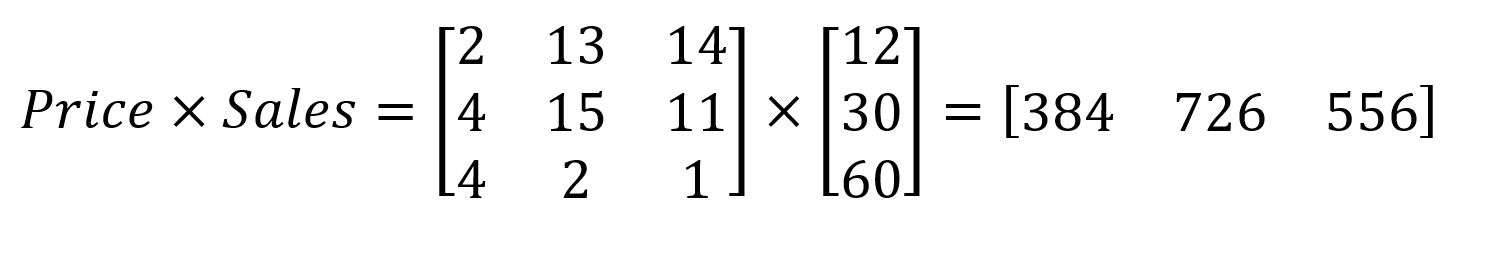Matrices and their operations also play a fundamental role in understanding the logic between arrays in programming. Matrices are stored as an array of arrays in programming. They are also referred to as multidimensional arrays.

Linear algebra is at the core of many advanced mathematical tools, such as the Fourier series and solving linear equations. It also plays a key role in other fields such as statistics.

## Quick overview of special matrices

Some matrices show up repeatedly throughout research and have been given particular names so they can be identified readily.

For example, the zero matrix simply has all values set to 0.The identity matrix is another special matrix. It is a square matrix where the number of columns and rows are equal. The diagonal values where “m” and “m” are equal are set to 1.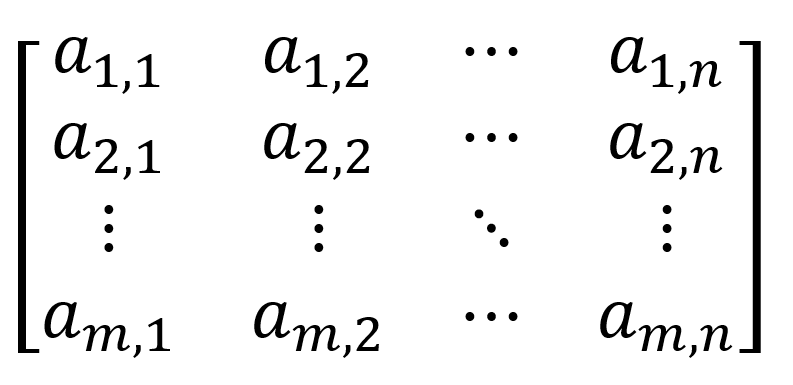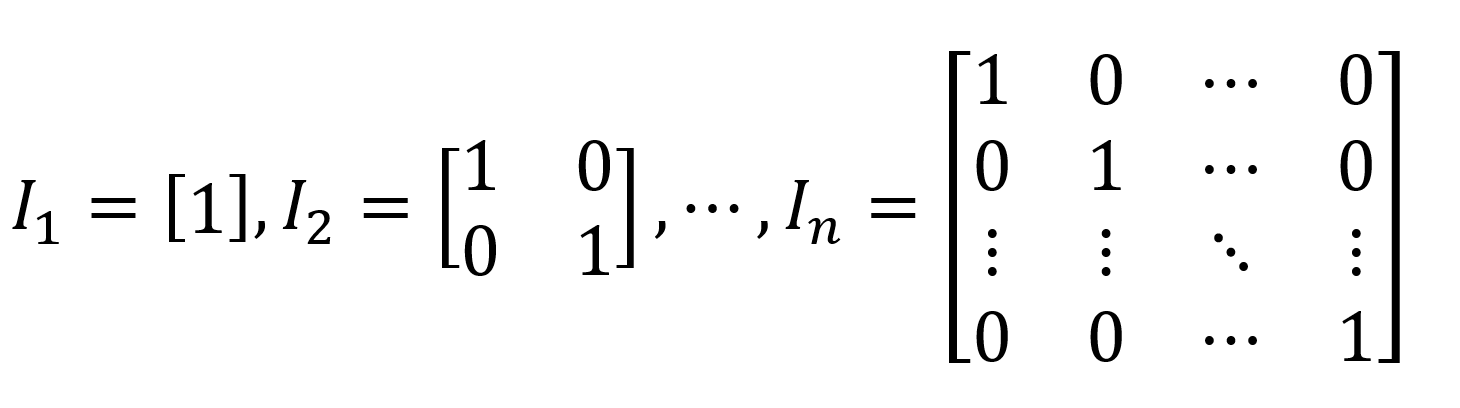The specific conditions for the identity matrix are denoted by: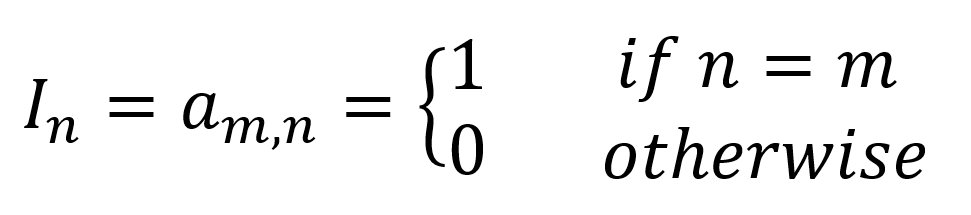A square matrix multiplied by an identity matrix will result in no change in the square matrix. For example: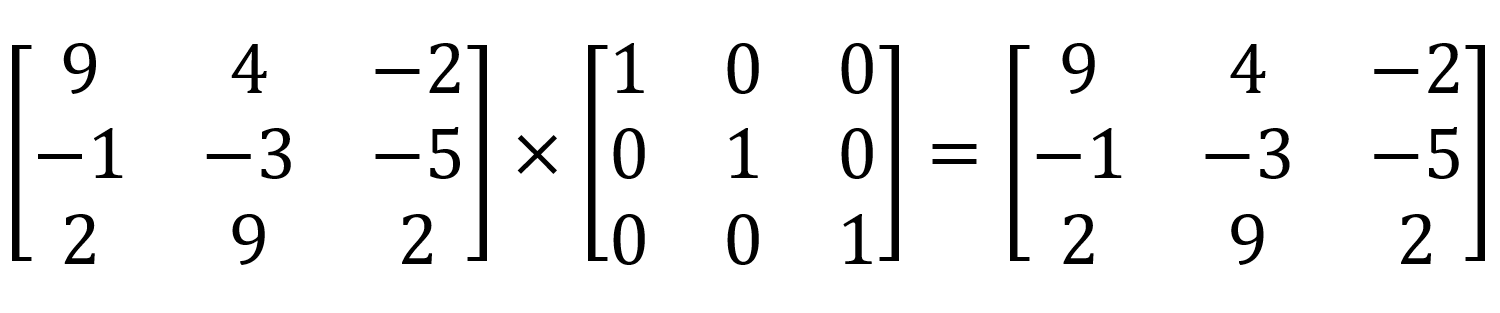To demonstrate, calculating the dot product of the first value uses the below equation:

c1,1=91 + 40 + (-2)0 = 9

## Keep practicing

Matrix multiplication is a foundational exercise in linear algebra and is a required course for most STEM majors. Mastering this operation allows students to have a deeper understanding of linear algebra.

Ensure you practice matrix multiplication by hand. It’s easy to overlook a number when calculating the dot product during an exam or test. Practice should help you mitigate the possibility of errors that could have been avoided were you more familiar with the method of calculation.

If you’re struggling with linear algebra, online courses will cover a variety of operations and linear transformations, so you’ll be ready for your exams. These courses introduce new notations and abstract ways of thinking that might be foreign to some students.

For one-on-one help with matrix multiplication, you can find the best tutors at HeyTutor.

### Top Posts

Education: College Majors with the Highest Salaries by Heytutor Blog
Education: States with the Largest Teacher Pay Gap by Heytutor Blog